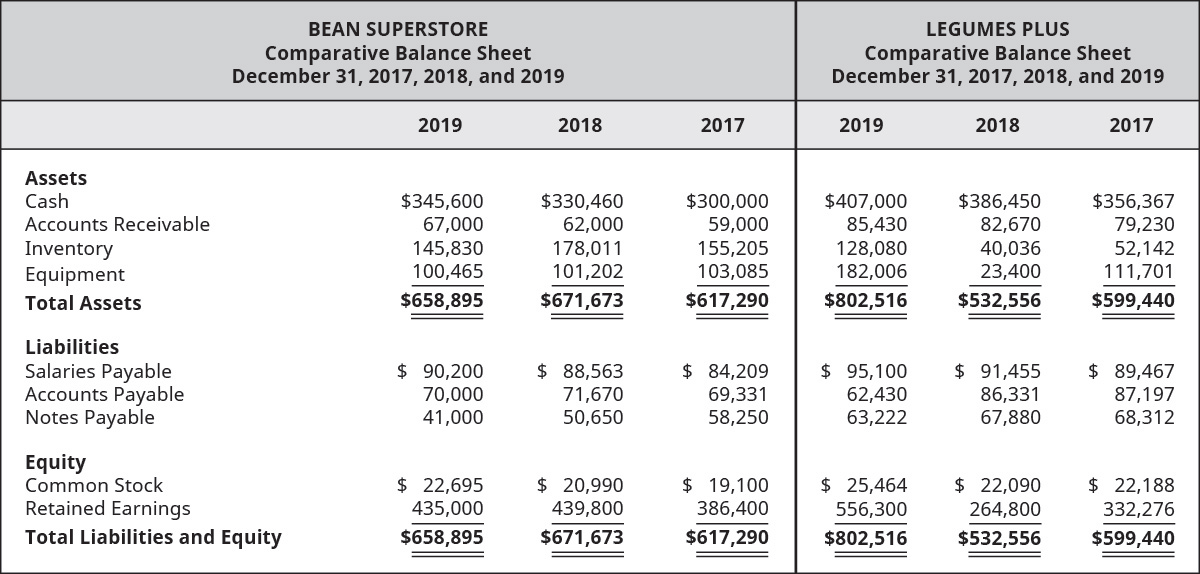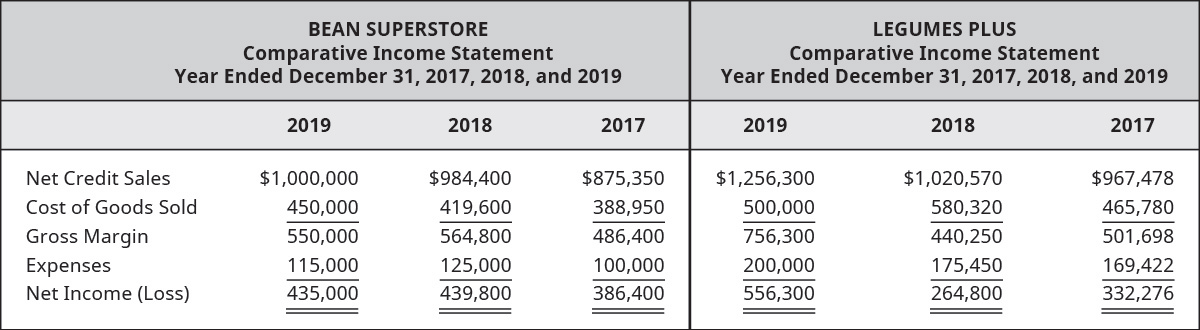# Review the select information for Bean Superstore and Legumes Plus (industry competitors), and then complete the following. A. Compute the accounts receivable turnover ratios for each company for 2018 and 2019. B. Compute the number of days’ sales in receivables ratios for each company for 2018 and 2019. C. Determine which company is the better investment and why. Round answers to two decimal places.FindFindarrow_forward

### Principles of Accounting Volume 1

19th Edition
OpenStax
Publisher: OpenStax College
ISBN: 9781947172685

#### Solutions

Chapter
SectionFindFindarrow_forward

### Principles of Accounting Volume 1

19th Edition
OpenStax
Publisher: OpenStax College
ISBN: 9781947172685
Chapter 9, Problem 7PA
Textbook Problem
1 views

## Review the select information for Bean Superstore and Legumes Plus (industry competitors), and then complete the following.A. Compute the accounts receivable turnover ratios for each company for 2018 and 2019.B. Compute the number of days’ sales in receivables ratios for each company for 2018 and 2019.C. Determine which company is the better investment and why. Round answers to two decimal places.To determine

(a)

Introduction:

The accounts receivable turnover ratio shows the company’s performance with respect to the company’s debt collection and company’s net credit sales. The number of days’ in receivable ratios shows the estimated days to convert accounts receivable into cash.

To calculate:

Account receivable turnover ratio of the companies for the year 2018 & 2019.

### Explanation of Solution

B Superstore:

Formula to calculate accounts receivable turnover ratio in 2018:

AccountsReceivablesTurnoverRatio=(NetCreditSalesAverageAccountsReceivables)

Substitute, $984,400 in net credit sales and$60,500 in average accounts receivables.

AccountsReceivablesTurnoverRatio=($984,400$60,5001)=16.27

Therefore, account receivable turnover ratio in 2018 is 16.27.

Formula to calculate accounts receivable turnover ratio in 2019:

AccountsReceivablesTurnoverRatio=(NetCreditSalesAverageAccountsReceivables)

Substitute, $1,000,000 in net credit sales and$64,500 in average accounts receivables.

AccountsReceivablesTurnoverRatio=($1,000,000$64,5002)=15.50

Therefore, account receivable turnover ratio in 2019 is 15.50.

L. Plus:

Formula to calculate accounts receivable turnover ratio in 2018:

AccountsReceivablesTurnoverRatio=(NetCreditSalesAverageAccountsReceivables)

Substitute, $1,020,570 in net credit sales and$80,950 in average accounts receivables.

AccountsReceivablesTurnoverRatio=($1,020,570$80,9503)=12.60

Therefore, account receivable turnover ratio in 2018 is 12

To determine

(b)

To calculate:

Number of days’ sales in receivable ratio of the companies for the year 2018 & 2019.

To determine

(c)

The company which is better among two stated.

### Still sussing out bartleby?

Check out a sample textbook solution.

See a sample solution

#### The Solution to Your Study Problems

Bartleby provides explanations to thousands of textbook problems written by our experts, many with advanced degrees!

Get Started

Find more solutions based on key concepts
Identify the five core objectives of the customer perspective.

Cornerstones of Cost Management (Cornerstones Series)

What are the three steps to follow in preparing a bank reconciliation?

College Accounting, Chapters 1-27 (New in Accounting from Heintz and Parry)

Explain the four types of corporate cultures.

Foundations of Business (MindTap Course List)

A life insurance policy is a financial asset, with the premiums paid representing the investments cost. a. How ...

Fundamentals of Financial Management, Concise Edition (with Thomson ONE - Business School Edition, 1 term (6 months) Printed Access Card) (MindTap Course List)